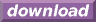#Calcute: freeware calculator

## Subtraction (sub, -)

A subtraction is the inverse of an addition. It is the combination of two numbers to produce their difference. Calcute implements it using either the sub function or, equivalently, the - (minus) operator.

```sub(44; 33)
11

44 - 33
11
```

In a subtraction, the number from which a value is being subtracted is called a "minuend". The value that is subtracted is called the subtrahend. The result of a subtraction is called the "difference".